Intermediate Algebra 2e

# 2.3Solve a Formula for a Specific Variable

Intermediate Algebra 2e2.3 Solve a Formula for a Specific Variable

### Learning Objectives

By the end of this section, you will be able to:

• Solve a formula for a specific variable
• Use formulas to solve geometry applications

### Be Prepared 2.7

Before you get started, take this readiness quiz.

Evaluate $2(x+3)2(x+3)$ when $x=5.x=5.$
If you missed this problem, review Example 1.6.

### Be Prepared 2.8

The length of a rectangle is three less than the width. Let w represent the width. Write an expression for the length of the rectangle.
If you missed this problem, review Example 1.10.

### Be Prepared 2.9

Evaluate $12bh12bh$ when $b=14b=14$ and $h=9.h=9.$
If you missed this problem, review Example 1.33.

### Solve a Formula for a Specific Variable

We have all probably worked with some geometric formulas in our study of mathematics. Formulas are used in so many fields, it is important to recognize formulas and be able to manipulate them easily.

It is often helpful to solve a formula for a specific variable. If you need to put a formula in a spreadsheet, it is not unusual to have to solve it for a specific variable first. We isolate that variable on one side of the equals sign with a coefficient of one and all other variables and constants are on the other side of the equal sign.

Geometric formulas often need to be solved for another variable, too. The formula $V=13πr2hV=13πr2h$ is used to find the volume of a right circular cone when given the radius of the base and height. In the next example, we will solve this formula for the height.

### Example 2.28

Solve the formula $V=13πr2hV=13πr2h$ for h.

### Try It 2.55

Use the formula $A=12bhA=12bh$ to solve for b.

### Try It 2.56

Use the formula $A=12bhA=12bh$ to solve for h.

In the sciences, we often need to change temperature from Fahrenheit to Celsius or vice versa. If you travel in a foreign country, you may want to change the Celsius temperature to the more familiar Fahrenheit temperature.

### Example 2.29

Solve the formula $C=59(F−32)C=59(F−32)$ for F.

### Try It 2.57

Solve the formula $F=95C+32F=95C+32$ for C.

### Try It 2.58

Solve the formula $A=12h(b+B)A=12h(b+B)$ for b.

The next example uses the formula for the surface area of a right cylinder.

### Example 2.30

Solve the formula $S=2πr2+2πrhS=2πr2+2πrh$ for h.

### Try It 2.59

Solve the formula $A=P+PrtA=P+Prt$ for t.

### Try It 2.60

Solve the formula $A=P+PrtA=P+Prt$ for r.

Sometimes we might be given an equation that is solved for y and need to solve it for x, or vice versa. In the following example, we’re given an equation with both x and y on the same side and we’ll solve it for y.

### Example 2.31

Solve the formula $8x+7y=158x+7y=15$ for y.

### Try It 2.61

Solve the formula $4x+7y=94x+7y=9$ for y.

### Try It 2.62

Solve the formula $5x+8y=15x+8y=1$ for y.

### Use Formulas to Solve Geometry Applications

In this objective we will use some common geometry formulas. We will adapt our problem solving strategy so that we can solve geometry applications. The geometry formula will name the variables and give us the equation to solve.

In addition, since these applications will all involve shapes of some sort, most people find it helpful to draw a figure and label it with the given information. We will include this in the first step of the problem solving strategy for geometry applications.

### How To

#### Solve geometry applications.

1. Step 1. Read the problem and make sure all the words and ideas are understood.
2. Step 2. Identify what you are looking for.
3. Step 3. Name what we are looking for by choosing a variable to represent it. Draw the figure and label it with the given information.
4. Step 4. Translate into an equation by writing the appropriate formula or model for the situation. Substitute in the given information.
5. Step 5. Solve the equation using good algebra techniques.
6. Step 6. Check the answer in the problem and make sure it makes sense.
7. Step 7. Answer the question with a complete sentence.

When we solve geometry applications, we often have to use some of the properties of the figures. We will review those properties as needed.

The next example involves the area of a triangle. The area of a triangle is one-half the base times the height. We can write this as $A=12bh,A=12bh,$ where b = length of the base and h = height.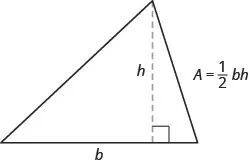### Example 2.32

The area of a triangular painting is 126 square inches. The base is 18 inches. What is the height?

### Try It 2.63

The area of a triangular church window is 90 square meters. The base of the window is 15 meters. What is the window’s height?

### Try It 2.64

A triangular tent door has an area of 15 square feet. The height is five feet. What is the length of the base?

In the next example, we will work with a right triangle. To solve for the measure of each angle, we need to use two triangle properties. In any triangle, the sum of the measures of the angles is $180°.180°.$ We can write this as a formula: $m∠A+m∠B+m∠C=180.m∠A+m∠B+m∠C=180.$ Also, since the triangle is a right triangle, we remember that a right triangle has one $90°90°$ angle.

Here, we will have to define one angle in terms of another. We will wait to draw the figure until we write expressions for all the angles we are looking for.

### Example 2.33

The measure of one angle of a right triangle is 40 degrees more than the measure of the smallest angle. Find the measures of all three angles.

### Try It 2.65

The measure of one angle of a right triangle is 50 more than the measure of the smallest angle. Find the measures of all three angles.

### Try It 2.66

The measure of one angle of a right triangle is 30 more than the measure of the smallest angle. Find the measures of all three angles.

The next example uses another important geometry formula. The Pythagorean Theorem tells how the lengths of the three sides of a right triangle relate to each other. Writing the formula in every exercise and saying it aloud as you write it may help you memorize the Pythagorean Theorem.

### The Pythagorean Theorem

In any right triangle, where a and b are the lengths of the legs, and c is the length of the hypotenuse, the sum of the squares of the lengths of the two legs equals the square of the length of the hypotenuse.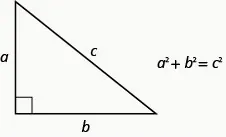We will use the Pythagorean Theorem in the next example.

### Example 2.34

Use the Pythagorean Theorem to find the length of the other leg in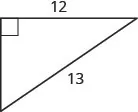### Try It 2.67

Use the Pythagorean Theorem to find the length of the leg in the figure.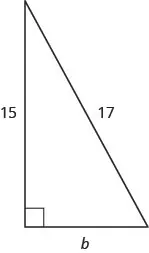### Try It 2.68

Use the Pythagorean Theorem to find the length of the leg in the figure.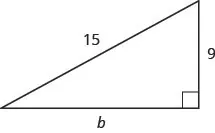The next example is about the perimeter of a rectangle. Since the perimeter is just the distance around the rectangle, we find the sum of the lengths of its four sides—the sum of two lengths and two widths. We can write is as $P=2L+2WP=2L+2W$ where L is the length and $WW$ is the width. To solve the example, we will need to define the length in terms of the width.

### Example 2.35

The length of a rectangle is six centimeters more than twice the width. The perimeter is 96 centimeters. Find the length and width.

### Try It 2.69

The length of a rectangle is seven more than twice the width. The perimeter is 110 inches. Find the length and width.

### Try It 2.70

The width of a rectangle is eight yards less than twice the length. The perimeter is 86 yards. Find the length and width.

The next example is about the perimeter of a triangle. Since the perimeter is just the distance around the triangle, we find the sum of the lengths of its three sides. We can write this as $P=a+b+c,P=a+b+c,$ where a, b, and c are the lengths of the sides.

### Example 2.36

One side of a triangle is three inches more than the first side. The third side is two inches more than twice the first. The perimeter is 29 inches. Find the length of the three sides of the triangle.

### Try It 2.71

One side of a triangle is seven inches more than the first side. The third side is four inches less than three times the first. The perimeter is 28 inches. Find the length of the three sides of the triangle.

### Try It 2.72

One side of a triangle is three feet less than the first side. The third side is five feet less than twice the first. The perimeter is 20 feet. Find the length of the three sides of the triangle.

### Example 2.37

The perimeter of a rectangular soccer field is 360 feet. The length is 40 feet more than the width. Find the length and width.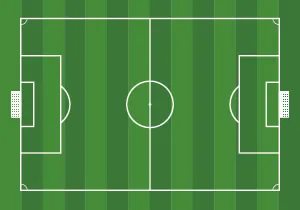### Try It 2.73

The perimeter of a rectangular swimming pool is 200 feet. The length is 40 feet more than the width. Find the length and width.

### Try It 2.74

The length of a rectangular garden is 30 yards more than the width. The perimeter is 300 yards. Find the length and width.

Applications of these geometric properties can be found in many everyday situations as shown in the next example.

### Example 2.38

Kelvin is building a gazebo and wants to brace each corner by placing a 10” piece of wood diagonally as shown.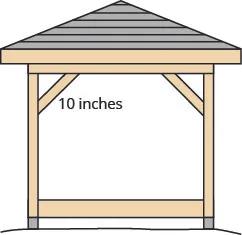How far from the corner should he fasten the wood if wants the distances from the corner to be equal? Approximate to the nearest tenth of an inch.

### Try It 2.75

John puts the base of a 13-foot ladder five feet from the wall of his house as shown in the figure. How far up the wall does the ladder reach?### Try It 2.76

Randy wants to attach a 17-foot string of lights to the top of the 15 foot mast of his sailboat, as shown in the figure. How far from the base of the mast should he attach the end of the light string?### Media

Access this online resource for additional instruction and practice with solving for a variable in literal equations.

### Section 2.3 Exercises

#### Practice Makes Perfect

Solve a Formula for a Specific Variable

In the following exercises, solve the given formula for the specified variable.

165.

Solve the formula $C=πdC=πd$ for d.

166.

Solve the formula $C=πdC=πd$ for $π.π.$

167.

Solve the formula $V=LWHV=LWH$ for L.

168.

Solve the formula $V=LWHV=LWH$ for H.

169.

Solve the formula $A=12bhA=12bh$ for b.

170.

Solve the formula $A=12bhA=12bh$ for h.

171.

Solve the formula
$A=12d1d2A=12d1d2$ for $d1.d1.$

172.

Solve the formula
$A=12d1d2A=12d1d2$ for $d2.d2.$

173.

Solve the formula
$A=12h(b1+b2)A=12h(b1+b2)$ for $b1.b1.$

174.

Solve the formula
$A=12h(b1+b2)A=12h(b1+b2)$ for $b2.b2.$

175.

Solve the formula
$h=54t+12at2h=54t+12at2$ for a.

176.

Solve the formula
$h=48t+12at2h=48t+12at2$ for a.

177.

Solve $180=a+b+c180=a+b+c$ for a.

178.

Solve $180=a+b+c180=a+b+c$ for c.

179.

Solve the formula
$A=12pl+BA=12pl+B$ for p.

180.

Solve the formula
$A=12pl+BA=12pl+B$ for l.

181.

Solve the formula
$P=2L+2WP=2L+2W$ for L.

182.

Solve the formula
$P=2L+2WP=2L+2W$ for W.

In the following exercises, solve for the formula for y.

183.

Solve the formula
$8x+y=158x+y=15$ for y.

184.

Solve the formula
$9x+y=139x+y=13$ for y.

185.

Solve the formula
$−4x+y=−6−4x+y=−6$ for y.

186.

Solve the formula
$−5x+y=−1−5x+y=−1$ for y.

187.

Solve the formula
$x−y=−4x−y=−4$ for y.

188.

Solve the formula
$x−y=−3x−y=−3$ for y.

189.

Solve the formula
$4x+3y=74x+3y=7$ for y.

190.

Solve the formula
$3x+2y=113x+2y=11$ for y.

191.

Solve the formula
$2x+3y=122x+3y=12$ for y.

192.

Solve the formula
$5x+2y=105x+2y=10$ for y.

193.

Solve the formula
$3x−2y=183x−2y=18$ for y.

194.

Solve the formula
$4x−3y=124x−3y=12$ for y.

Use Formulas to Solve Geometry Applications

In the following exercises, solve using a geometry formula.

195.

A triangular flag has area 0.75 square feet and height 1.5 foot. What is its base?

196.

A triangular window has area 24 square feet and height six feet. What is its base?

197.

What is the base of a triangle with area 207 square inches and height 18 inches?

198.

What is the height of a triangle with area 893 square inches and base 38 inches?

199.

The two smaller angles of a right triangle have equal measures. Find the measures of all three angles.

200.

The measure of the smallest angle of a right triangle is $20°20°$ less than the measure of the next larger angle. Find the measures of all three angles.

201.

The angles in a triangle are such that one angle is twice the smallest angle, while the third angle is three times as large as the smallest angle. Find the measures of all three angles.

202.

The angles in a triangle are such that one angle is 20 more than the smallest angle, while the third angle is three times as large as the smallest angle. Find the measures of all three angles.

In the following exercises, use the Pythagorean Theorem to find the length of the hypotenuse.

203.204.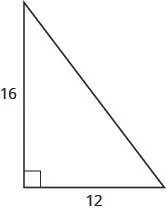205.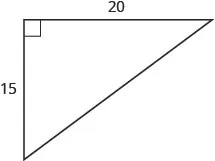206.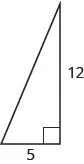In the following exercises, use the Pythagorean Theorem to find the length of the leg. Round to the nearest tenth if necessary.

207.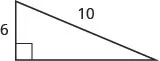208.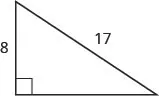209.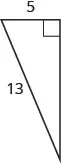210.211.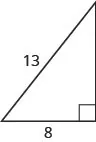212.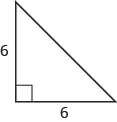213.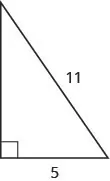214.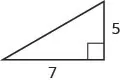In the following exercises, solve using a geometry formula.

215.

The width of a rectangle is seven meters less than the length. The perimeter is 58 meters. Find the length and width.

216.

The length of a rectangle is eight feet more than the width. The perimeter is 60 feet. Find the length and width.

217.

The width of the rectangle is 0.7 meters less than the length. The perimeter of a rectangle is 52.6 meters. Find the dimensions of the rectangle.

218.

The length of the rectangle is 1.1 meters less than the width. The perimeter of a rectangle is 49.4 meters. Find the dimensions of the rectangle.

219.

The perimeter of a rectangle of 150 feet. The length of the rectangle is twice the width. Find the length and width of the rectangle.

220.

The length of the rectangle is three times the width. The perimeter of a rectangle is 72 feet. Find the length and width of the rectangle.

221.

The length of the rectangle is three meters less than twice the width. The perimeter of a rectangle is 36 meters. Find the dimensions of the rectangle.

222.

The length of a rectangle is five inches more than twice the width. The perimeter is 34 inches. Find the length and width.

223.

The perimeter of a triangle is 39 feet. One side of the triangle is one foot longer than the second side. The third side is two feet longer than the second side. Find the length of each side.

224.

The perimeter of a triangle is 35 feet. One side of the triangle is five feet longer than the second side. The third side is three feet longer than the second side. Find the length of each side.

225.

One side of a triangle is twice the smallest side. The third side is five feet more than the shortest side. The perimeter is 17 feet. Find the lengths of all three sides.

226.

One side of a triangle is three times the smallest side. The third side is three feet more than the shortest side. The perimeter is 13 feet. Find the lengths of all three sides.

227.

The perimeter of a rectangular field is 560 yards. The length is 40 yards more than the width. Find the length and width of the field.

228.

The perimeter of a rectangular atrium is 160 feet. The length is 16 feet more than the width. Find the length and width of the atrium.

229.

A rectangular parking lot has perimeter 250 feet. The length is five feet more than twice the width. Find the length and width of the parking lot.

230.

A rectangular rug has perimeter 240 inches. The length is 12 inches more than twice the width. Find the length and width of the rug.

In the following exercises, solve. Approximate answers to the nearest tenth, if necessary.

231.

A 13-foot string of lights will be attached to the top of a 12-foot pole for a holiday display as shown. How far from the base of the pole should the end of the string of lights be anchored?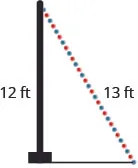232.

am wants to put a banner across her garage door diagonally, as shown, to congratulate her son for his college graduation. The garage door is 12 feet high and 16 feet wide. Approximately how long should the banner be to fit the garage door?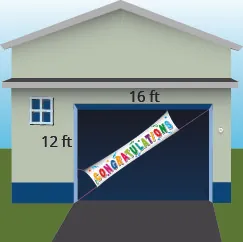233.

Chi is planning to put a diagonal path of paving stones through her flower garden as shown. The flower garden is a square with side 10 feet. What will the length of the path be?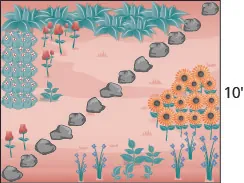234.

Brian borrowed a 20-foot extension ladder to use when he paints his house. If he sets the base of the ladder six feet from the house as shown, how far up will the top of the ladder reach?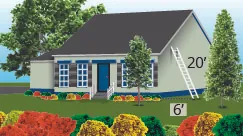#### Everyday Math

235.

Converting temperature While on a tour in Greece, Tatyana saw that the temperature was 40° Celsius. Solve for F in the formula $C=59(F−32)C=59(F−32)$ to find the Fahrenheit temperature.

236.

Converting temperature Yon was visiting the United States and he saw that the temperature in Seattle one day was 50° Fahrenheit. Solve for C in the formula $F=95C+32F=95C+32$ to find the Celsius temperature.

237.

Christa wants to put a fence around her triangular flowerbed. The sides of the flowerbed are six feet, eight feet and 10 feet. How many feet of fencing will she need to enclose her flowerbed?

238.

Jose just removed the children’s play set from his back yard to make room for a rectangular garden. He wants to put a fence around the garden to keep the dog out. He has a 50-foot roll of fence in his garage that he plans to use. To fit in the backyard, the width of the garden must be 10 feet. How long can he make the other side?

#### Writing Exercises

239.

If you need to put tile on your kitchen floor, do you need to know the perimeter or the area of the kitchen? Explain your reasoning.

240.

If you need to put a fence around your backyard, do you need to know the perimeter or the area of the backyard? Explain your reasoning.

241.

Look at the two figures below.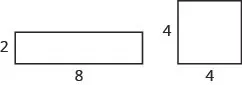Which figure looks like it has the larger area? Which looks like it has the larger perimeter?
Now calculate the area and perimeter of each figure. Which has the larger area? Which has the larger perimeter?
Were the results of part (b) the same as your answers in part (a)? Is that surprising to you?

242.

Write a geometry word problem that relates to your life experience, then solve it and explain all your steps.

#### Self Check

After completing the exercises, use this checklist to evaluate your mastery of the objectives of this section.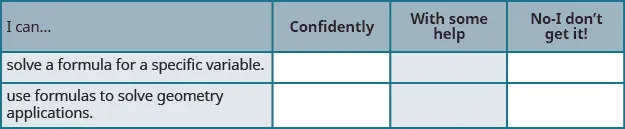What does this checklist tell you about your mastery of this section? What steps will you take to improve?

Order a print copy

As an Amazon Associate we earn from qualifying purchases.